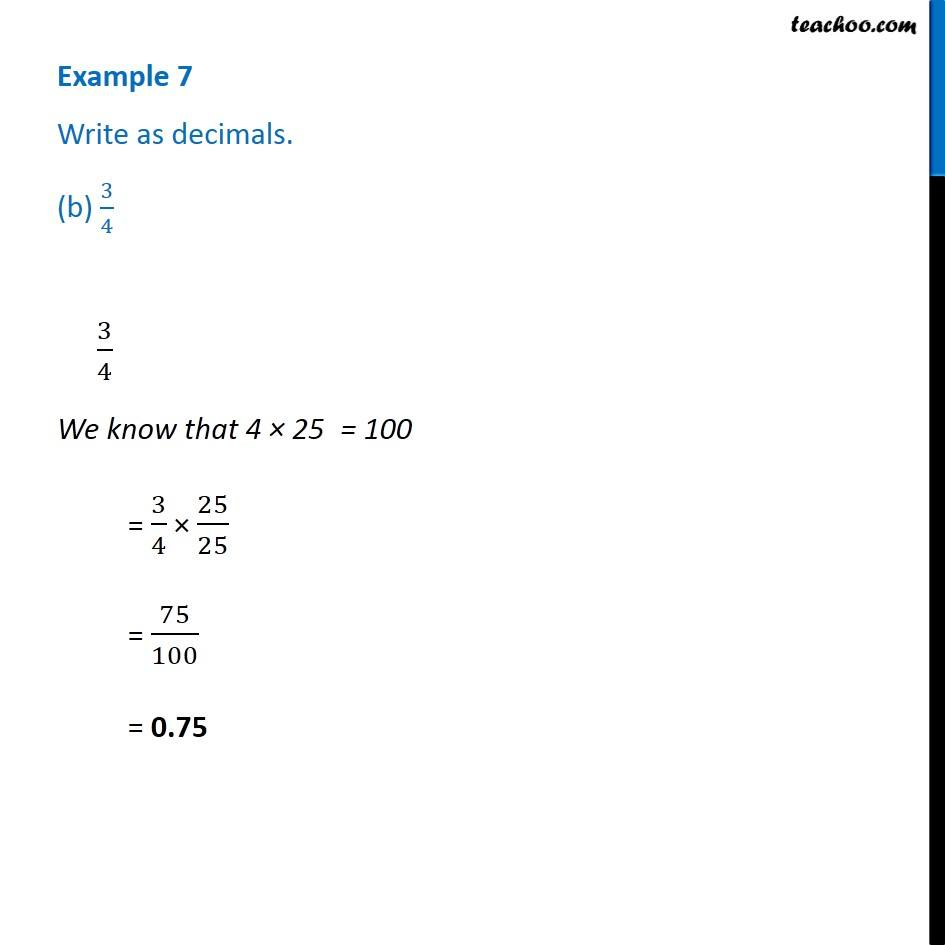1. Chapter 8 Class 6 Decimals
2. Serial order wise
3. Examples

Transcript

Example 7 Write as decimals. (c) 3/4 = 3/4 We know that 4x25=100 = 3/4 x 25/25 = 75/100 = 0.75